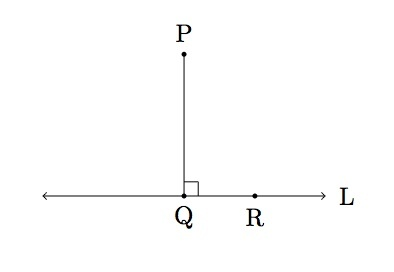# Shortest line segment from a point $P$ to a line $L$

Alignments to Content Standards: G-SRT.C.8

Suppose $P$ is a point not contained on a line $L$. Let $Q$ be the point on $L$ so that $PQ$ meets $L$ perpendicularly and let $R$ be any other point on $L$ as in the picture below:Show that segment $PQ$ is shorter than segment $PR$.

## IM Commentary

This is a foundational geometry task designed to provide a route for students to develop some fundamental geometric properties that may seem rather obvious at first glance. In this case, the fundamental property in question is that the shortest path from a point to a line meets the line at a right angle, which is crucial for many further developments in the subject. As such, teachers electing to have students justify this result should make it clear which existing properties are available for use. In the solution provided, a very brief proof is given which only relies on the Pythagorean Theorem. It is probably worth remarking that the Pythagorean Theorem is the cornerstone for evaluating the distance between two points, and here is used to further solve the question of the distance between a point and a line.

This task can be used for instruction or for assessment. If it is used for instruction, the teacher may want to have the students supply the picture. The task should be done relatively early within a geometry course because of its intuitive appeal. In fact, an ideal time to cover this task is at the same time that the notion of sraightline distance in the plane is introduced. Since the result is so immediately plausible, it seems reasonable to use this task for assessment purposes only in classes where providing proofs of such statements is a regular occurrence.

Finally, we comment on alignment: The standard G-SRT.8 invokes using the Pythagorean Theorem to solve applied problems. While the current task is not an "applied problem" in the sense of physics and engineering, it gives a nice example of applying one major result in mathematics in order to deduce further mathematics.

## Solution

Below is a picture of the segments $PQ$ and $PR$ which need to be compared:The picture makes it clear that segment $PR$ is longer than segment $PQ$. To formulate an argument, we need to quantify the length of segment $PR$ in terms of the length of segment $PQ$. Since triangle $PQR$ is a right triangle and side $PR$ is opposite the right angle, the Pythagorean theorem tells us that $$|PR|^2 = |PQ|^2 + |QR|^2.$$ Since $|QR| \gt 0$ we have $$|PR|^2 \gt |PQ|^2$$ and this implies that $|PR| \gt |PQ|$. So segment $PQ$ is shorter than segment $PR$ as desired.